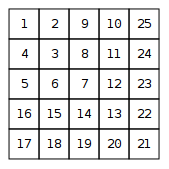CSES - Number Spiral
• Time limit: 1.00 s
• Memory limit: 512 MB

A number spiral is an infinite grid whose upper-left square has number 1. Here are the first five layers of the spiral:Your task is to find out the number in row y and column x.

# Input

The first input line contains an integer t: the number of tests.

After this, there are t lines, each containing integers y and x.

# Output

For each test, print the number in row y and column x.

# Constraints

• 1 \le t \le 10^5
• 1 \le y,x \le 10^9

# Example

Input:

3
2 3
1 1
4 2


Output:

8
1
15